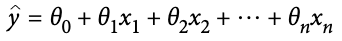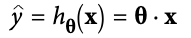top of page
Search
• Divakar V

# H.O.M.L Ch-4 | Training models algo

Updated: May 9, 2021

Uncovering some of the black box algorithms in this chapter. Mainly we'll be talking about Linear Regression, Polynomial Regression, Logistic Regression and Softmax Regression.

Contents:

1. Linear Regression

2. Direct approach - "closed-form" / Normal equation

3. Iterative approach - Gradient Descent

4. Polynomial Regression - fitting nonlinear dataset

5. Logistic Regression

6. Softmax Regression

## Linear Regression

A linear model makes a prediction by simply computing a weighted sum of input features, plus a constant called the bias term (or the intercept term).The aim is to find the best fitting model on the training set by finding the optimal values of the parameters (𝜃). Root Mean Square Error (RMSE) is a common performance measure for regression models.

### 1. Normal Equation

One way to find the optimal ø value that minimized the cost function is to use a closed-form equation. In other words, use a mathematical equation that gives the direct result.

This generally involves computing the inverse of matrices.The pseudo-inverse itself is computed using a standard matrix factorization technique - Singular Value Decomposition (SVD)

Computational Complexity:

• SVD approach is more efficient than computing Normal Equation.

• SVD approach handles edge cases. Normal equation may not work if XTX is not invertible. Cases where m<n.

• Normal eq. computes inverse of XTX which is of size O(n^2). Complexity of inverting such a matrix is typically about O(n^2.4) to O(n^3).

• Complexity of SVD is O(n^2)

• However, both approaches (normal & svd) get very slow when number of features (n) grows large (e.g. 100,000).

• On the positive side, both are linear w.r.t number of training instances (m) i.e. O(m). Hence, both can handle large no. of training instances provided they fit in memory!

• Prediction complexity - both approaches are linear w.r.t m and n, hence, fast.

• Drawback of this method - Not suited for situations when

• No. of features become very large

• Too many training instances to fit into the memory.

This is a generic optimization algorithm which is capable of finding optimal solution to a wide range of problems.

Idea is to tweak parameters iteratively in order to minimize a cost function.2. Take incremental steps in the direction of descending slope trying to minimize cost function.

3. Repeat until it converges to a minimum.

4. An important parameter is the Learning Rate which determines the step size.

5. Too small learning rate - will take too much time to converge.

6. Too large learning rate - you might jump across the valley and end up higher than the starting value.

Not all cost functions look like nice regular bowls!

In reality, cost functions may be full of irregular terrains - holes, ridges, plateaus etc. This makes it difficult to converge to a minima. Hence, it can be quite challenging to make the algorithm converge to a global minima itself.

Fortunately, MSE cost function for linear regression happens to be a Convex function i.e. if you pick any 2 points on the curve, the line segment joining them never crosses the curve. So, there are no local minima, just one global minimum. It is also a continuous function & slope never changes abruptly. So, in this case, Gradient Descent is guaranteed to reach global minimum (if you train long enough & lr is not too high)

Note: Shape of the bowl depends on the scale of features also. So, better to bring all the features to a similar scale.

Often the learning algorithm tries to optimize a different function than the performance measure used for evaluation. This is generally because that learning algorithm is easier to compute, has nice differential properties or maybe because we want to constrain the model during training (regularization).

Implementing Gradient Descent requires computing gradients of the cost function. Depending upon the number of instances used to calculate gradients & update the weights, we have 3 types of algorithms:

1. Batch Gradient Descent - Compute gradients on the entire input set (whole batch). [Poor choice of name]

2. Stochastic Gradient Descent - Pick random instance from the training set and compute gradient on this single instance at a time. Do shuffle the instances.

3. Mini-batch Gradient Descent - Take a bunch of instances (neither the whole set nor a single instance) to compute gradients.

When dealing with a large number of input Features, use Gradient Descent over Normal Equation or SVD decomposition! It scales better.

Batch Gradient Descent - Not practical to use when laaarge number of training instances.

Stochastic Gradient Descent - Very irregular convergence. But if the cost function is very irregular, it can help to jump out of the local minima and have a better chance to converge to global minima.

Mini-batch Gradient Descent - less time to converge w.r.t Batch Gradient Descent. Less erratic w.r.t Stochastic Gradient Descent.

Batch G.D. - Actually stops at the minima

Stochastic & Mini-bathc G.D. - continue to walk around (jumping) near the minima

Till now we were looking at Linear Data. Moving on...

## Polynomial Regression

>>>>>>>> Post In Progress ...<<<<<<<<

See All
bottom of page